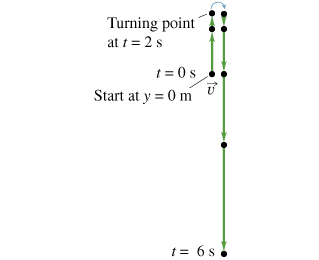# Problem: A stone is thrown upward from the edge of a cliff, reaches its maximum height, and then falls down into the valley below. A motion diagram for this situation is given (Figure 1) , beginning the instant the stone leaves the thrower’s hand.Construct a graph corresponding to the stone's vertical displacement, y(t), taking the acceleration due to gravity as exactly 10 m/s2. Ignore air resistance. The unit of time is in seconds and the unit of displacement is in meters. In plotting the points, round-off the coordinate values to the nearest integer.

###### FREE Expert Solution

UAM expressions:

Solve for V0 — consider from t = 0s to the turning point at t = 2 s:

vf = v0 - gt

vf = 0 m/s, g = 10 m/s2

v0 = vf + gt = 0 + (10)(2) = 20 m/s

Graphing:

81% (201 ratings)###### Problem Details

A stone is thrown upward from the edge of a cliff, reaches its maximum height, and then falls down into the valley below. A motion diagram for this situation is given (Figure 1) , beginning the instant the stone leaves the thrower’s hand.

Construct a graph corresponding to the stone's vertical displacement, y(t), taking the acceleration due to gravity as exactly 10 m/s2. Ignore air resistance. The unit of time is in seconds and the unit of displacement is in meters. In plotting the points, round-off the coordinate values to the nearest integer.Frequently Asked Questions

What scientific concept do you need to know in order to solve this problem?

Our tutors have indicated that to solve this problem you will need to apply the Duplicate concept. You can view video lessons to learn Duplicate. Or if you need more Duplicate practice, you can also practice Duplicate practice problems.